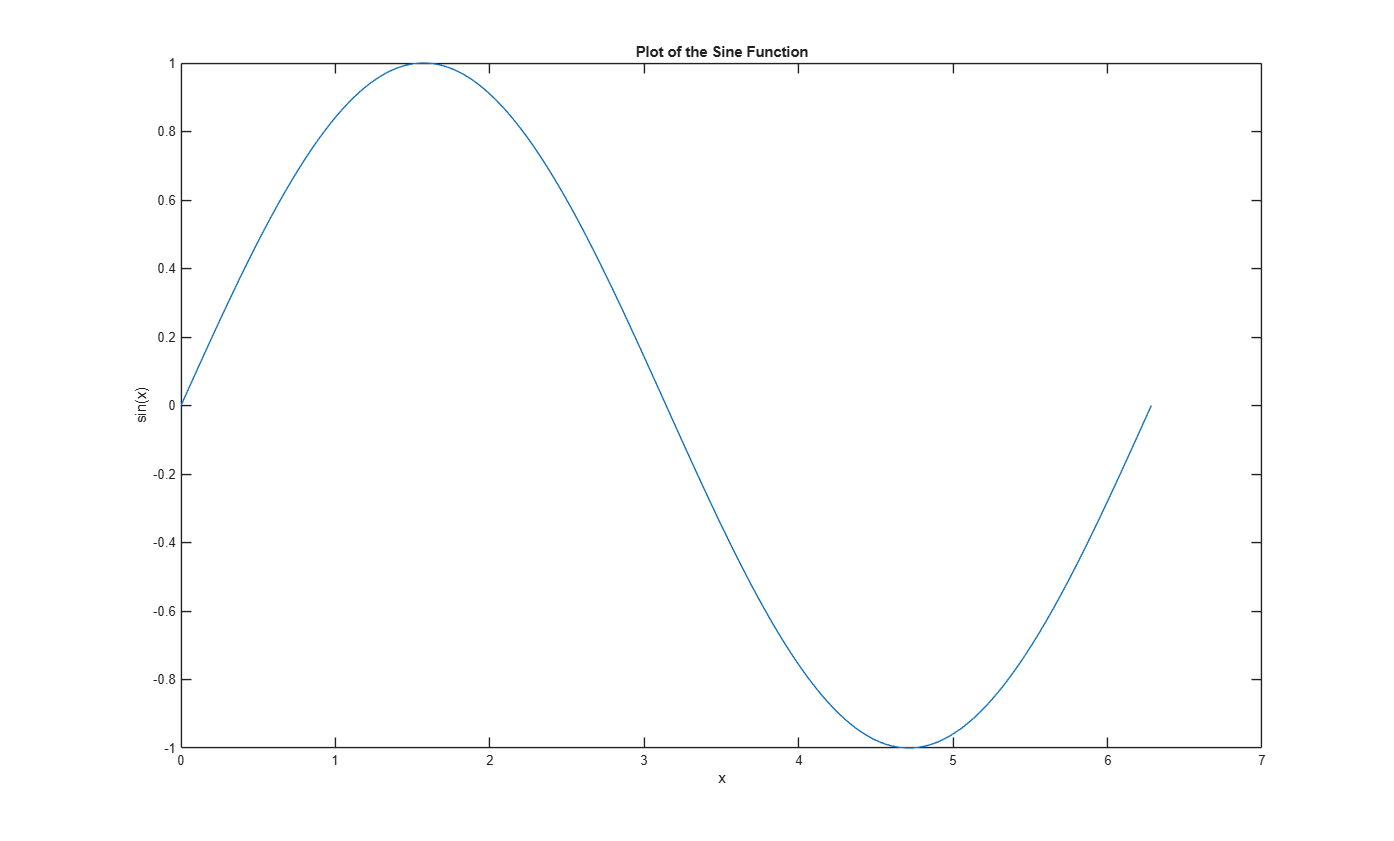Documentation

## Creating 2-D Plots

This example shows how to create 2-D line plots in MATLAB using the plot function.

Create a regularly-spaced vector x from 0 to 2*pi using pi/100 as the increment between elements.

```x = 0:pi/100:2*pi; ```

Calculate sine for each value in x.

```y = sin(x); ```

Use the figure command to create a new figure and plot command to display result.

```figure; plot(x, y) ```Label the axes and add a title so that viewers understand the purpose of your graph.

```xlabel('x') ylabel('sin(x)') title('Plot of the Sine Function') ```You can plot the same variables with specified line style, color, and marker (A marker is a symbol that appears at each plotted data point, such as a +, o, or *). Use 'help plot' to learn more about line specification.

In this example, the 'r--' string is a red dashed-line specification.

```figure plot(x, y, 'r--') title('Plot of the Sine Function (Red Dashed-line)') ```To add plots to an existing figure, use hold.

```figure plot(x, y) hold on y2 = cos(x); plot(x, y2, ':') legend('sin', 'cos') title('Plot of Sine and Cosine Functions') ```Use 'hold off' to stop plotting on top of the existing figure.

```hold off ```